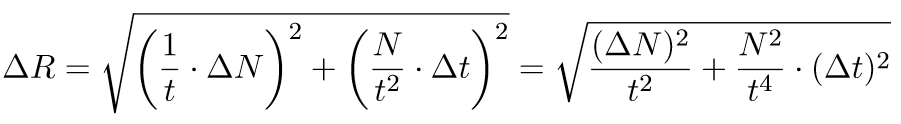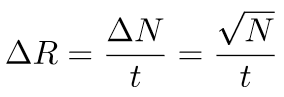# Glossar

All · A · C · D · E · F · G · L · M · N · P · S · T · W · Z

# E

## Error Calculation

In an experiment, the result of each measurement is slightly different due to measurement errors. Each measurement result is therefore only an approximation of the true value, or in other words: the measured value is the true, desired value with a certain error. To be able to work reasonably with the measurement results, this error should be as small as possible, the measurement result should be as close as possible to the true value.

The student experiments at Cosmic@Web measure the rate of cosmic particles $R$, i.e., the number of measured particles $N$ per time unit $t$:Because the rate is therefore dependent on $N$ and $t$, the error propagation law is used to calculate the measurement error $\Delta R$ (more information on this in the Wikipedia article). The formula for two independent variables, such as $N$ and $t$, is as follows:is the derivative of the functionwith respect to $N$, which is simply. Analogously,is the derivative with respect to $t$, it is. That's what we use and maintain:Because the computer measures time accurately by machine, the time deviation $\Delta t$ is very small. Accordingly, the square of it $\left(\Delta t\right)$2 is almost 0 and thus negligible. It remainsNow we still have to determine $\Delta N$. Natural processes like the cosmic particles showering down can be described very well with the Poisson or also Gaussian distribution. According to these mathematical laws, we can usefor the deviation. Now we know how big the deviation of the measured rate is from the true value:From this you can also determine how long you have to measure to stay below a certain error. The measurement deviation $\Delta R$ is the inaccuracy $x$ (in percent) from the true value $R$:This formula can now be simply solved for $x$. We getFinally, the time is determined:By using the expected rate for $R$ and a certain error, which you want to achieve, for $x$, you can calculate the necessary measurement duration $t$. Generally, it always makes sense to measure until the error is less than 1%. How many seconds would you have to measure if a rate of 6.67 Hz is expected? Remember that the unit Hz=1/s and 1%=0.01.

With the angle measurement one calculates in a similar way. Cosmic particles reach us from all directions. However, the rate we expect for the individual directions is different. The rate depends on the angle $\alpha$, in which one measures. Therefore the formula for the calculation of the rate at the angle measurement looks as follows:Because we simply lay the CosMO detector flat on the table for every other measurement, the measurement angle is then $\alpha$=0. With cos(0)=1, this gives exactly the simplified formula we used:In the case of angular measurement, the rate $R$ therefore depends on three variables which are independent of each other: the number $N$, the time $t$ and the angle $\alpha$. This time the formula is accordingly:Again, we neglect the summand with the time because it becomes almost 0. In the same way, the angle change is almost 0, so that this term also does not need to be considered further. The measurement deviation is here also:The inaccuracy in percent is then as follows with the slightly different formula for the rate:and the time to measure at an angle for a given error is obtained by substituting the values for the rate $R$, the error $x$ and the measurement angle $\alpha$ into the formula converted to $t$:## Errors

Systematic Errors
Systematic errors are caused by the measuring apparatus itself. The measurement result is distorted in the same direction every time the measurement is taken. Causes can be, for example, incorrect calibration of the measuring instruments, incorrect measuring set-ups or failure to take account of external influences such as voltage, temperature or air pressure fluctuations. The limited accuracy of measuring instruments must also be considered a systematic error. Such errors can only be detected and avoided by great care and experience of the experimenting person.

Statistical Errors
When measuring one and the same physical quantity, the same values do not result in the same values for each measurement. They fluctuate or scatter around a mean value. Unlike the systematic errors, the statistical errors do not always falsify the measurement result in the same direction. They are caused, for example, by inaccurate reading from the measuring instrument, by vibrations of the measuring instrument or by fluctuations of the quantity to be measured itself. Such errors can only be determined and corrected by mathematical methods.

For more detailed information see the definition and description of Wolfram Experimental Data Analyst Documentation.

## Exponential Functions

Exponential functions are mainly used to model percentage increases or decreases, multiplication times or half-lives. The general form is

$f\left(x\right)=a\cdot bx$

The parameter $a$ influences the slope and curvature of the graph and specifies the point of intersection with the y-axis (if the graph has not been moved along the x- and y-axis).
For $\mathrm{b > 1}$ the curve is monotonically rising, for $\mathrm{0 < b < 1}$ monotonically falling.

A special case is the natural or e-function $ex$, which is also displayed as  exp(x) .